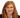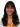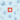TechnologyJun 04, 2014

# Twitter Analytics Using R Part 3: Compare SentimentsWe’ve been exploring the power of the programming language R for data mining. In this post we will analyze tweets from game two of the NBA playoffs series between the San Antonio Spurs and the Oklahoma City Thunder and create a graph comparing the average sentiment score during the game. Sentiment analyses classify communications as positive, negative, or neutral.

Determining sentiment ranges from very simple classification methods to very complex algorithms. For ease and transparency in this example, we will classify the sentiment of a tweet based on the polarity of the individual words. Each word will be given a score of +1 if classified as positive, -1 if negative, and 0 if classified as neutral. This will be determined using positive and negative lexicon lists compiled by Minqing Hu and Bing Liu for their work “Mining and Summarizing Customer Reviews.” The total polarity score of a given tweet will result in adding together the scores of all the individual words. Below is an example of a tweet that may be found in Twitter data for the Spurs.

RT @BooshBush: The energy in this place is INSANE! @JMV1070

Using the scoring system provided above, we would score the individuals words as follows:

− Neutral (0): RT, The, energy, in, this, place, is

− Negative (-1): insane

− Positive (+1): N/A

Adding together the scores of the individual words gives this tweet a total score of -1.

This works as a simple example of how to calculate the polarity score, though clearly it’s not very accurate. The algorithm misses out on the overall context of the tweet because it focuses on individual words. This could be corrected using more complex sentiment scoring algorithms and taking context into account. But we’ll still use it as a simple example to demonstrate the capabilities of R using social media data.

We will reuse the Twitter app we created and authorized in the preceding post, Twitter Analytics Using R Part 1: Extract Tweets to extract tweets for @Spurs and @OKCThunder. For our purposes we created a script, ExtractTweets.R, to pull data from Twitter for the duration of the game and saved all unique tweets in a file for our analysis.

Before we run the sentiment analysis, we need to load the R packages required for processing tweet strings and graphing the data. We then need to load the script file that contains the above-mentioned sentiment scoring. Additionally, we need to load the positive and negative lexicons that we will use to score each word. If a word is not included in either list, it will be classified as neutral. Finally, we need to read in the data previously saved from our tweet extraction script.

##### Install packages for sentiment analysis

install.packages("ggplot2") install.packages("plyr") install.packages("gridExtra") library("ggplot2") library("plyr") library("gridExtra")

##### algorithm described in this post

source("ScoreSentiment.R")

##### Load positive and negative lexicon files used to score individual words

pos = scan(file="positive-words.txt",what="charcter", comment.char=";") neg = scan(file="negative-words.txt",what="charcter", comment.char=";")

#read tweets into data frame from file Spursdf OKCThunderdf

### 2. Score Tweets

Now we proceed to score each extracted tweet using score.sentiment function. This function expects the tweet text and the positive and negative lexicons as inputs.

##### Score all the tweets for each team using the score.sentiment function available in the ScoreSentiment.R file

Spurs <- score.sentiment(Spursdf\$text, Spursdf\$created, pos,neg) OKCThunder <- score.sentiment(OKCThunderdf\$text, OKCThunderdf\$created, pos,neg)

### 3. Change Time Zone

The extracted tweets have a field (created) that shows the date timestamp of the tweets in UTC time zone. To improve readability, we will change the time zone to CST.

#change format of timestamp to CST Spurs\$created <- format(Spurs\$created,tz="America/Chicago") OKCThunder\$created <- format(OKCThunder\$created,tz="America/Chicago")

### 4. Summarize Data

Before plotting the scores on the graph, we will summarize the tweet scores by minute. Here we are using ddply from the plyr package to aggregate the tweets by minute and calculate the average score.

#group by hour, minute Spurs\$hour <- as.POSIXlt(Spurs\$created)\$hour Spurs\$min <- as.POSIXlt(Spurs\$created)\$min OKCThunder\$hour <- as.POSIXlt(OKCThunder\$created)\$hour OKCThunder\$min <- as.POSIXlt(OKCThunder\$created)\$min

#summary Spurs.summary <- ddply(Spurs, c("hour","min"), summarise, N = length(score), avg = mean(score)) Spurs.summary\$created <-as.POSIXct(factor(paste0(as.character(Spurs.summary\$hour),':',as.character(Spurs.summary\$min))) , format="%H:%M")

OKCThunder.summary <- ddply(OKCThunder, c("hour","min"), summarise, N = length(score), avg = mean(score)) OKCThunder.summary\$created <-as.POSIXct(factor(paste0(as.character(OKCThunder.summary\$hour), ':',as.character(OKCThunder.summary\$min))) , format="%H:%M")

### 5. Create the Grid

Now that we have extracted and scored the tweets for each team, we want to graph the results. Here we will use a line graph to display the results. The y-axis displays the average sentiment score of tweets. The x-axis shows the time the tweet was created. The two plots are arranged on a grid using the gridExtra package. The legend on the side helps map time to game events.

#plot by time and average score plot.OKCThunder <- ggplot(OKCThunder.summary, aes(x=created, y=avg))+ geom_line(color='blue')+ scale_x_datetime(limits = c(as.POSIXct(strptime("2014-05-22 20:00", "%Y-%m-%d %H:%M")), as.POSIXct(strptime("2014-05-22 22:30", "%Y-%m-%d %H:%M")))) + labs(title = "OKC Thunder", x = "Time", y = "Average Sentiment Score") + ylim(-1, 2) +theme_bw()

plot.Spurs <- ggplot(Spurs.summary, aes(x=created, y=avg))+ geom_line(color='slategray')+ scale_x_datetime(limits = c(as.POSIXct(strptime("2014-05-22 20:00", "%Y-%m-%d %H:%M")), as.POSIXct(strptime("2014-05-22 22:30", "%Y-%m-%d %H:%M")))) + labs(title = "Spurs", x = "Time", y = "Average Sentiment Score") + ylim(-1, 2) + theme_bw()

#create legend for grid legendtable <- data.frame(Time = c('20:00', '21:10', '21:30', '22:30'), Event = c("Tip Off","Half Time", "Third Quarter","Final Buzzer"))

legend <- tableGrob(legendtable,show.rowname=FALSE,gpar.coretext=gpar(fontsize=9), gpar.corefill = gpar(fill = "white", col = "grey95"), gpar.coltext=gpar(fontsize=9,fontface='bold'))

#arrange plots on grid grid.arrange(plot.Spurs, plot.OKCThunder, ncol=1, nrow=2, main="Spurs vs. OKC Thunder",legend=legend)

### Summary

Sentiment analysis allows organizations to quantify perceptions. If we look at the two graphs, both teams have positive tweets at the beginning of the game and continue until halftime. After halftime, we see an increase in positive tweets for the Spurs and an inverse trend for Thunder matching the outcome of the game (112-77).

The examples in this series are a brief introduction into the many things you can do with R. It has many strengths in statistical analysis and is a very powerful tool in the hands of a data miner. A wide variety of companies are using R daily, including Google, Bank of America, the InterContinental Hotels Group, and Shell.